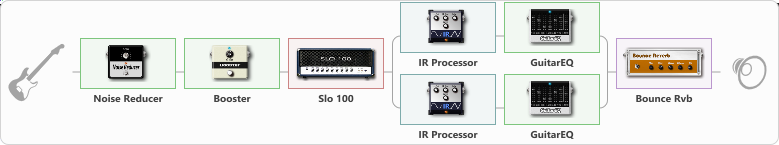# Rustam

Discussion in 'ToneLib-GFX presets' started by Assymmetry, Mar 6, 2023.

1. Rustam

Effects chain:Effect: "Noise Reducer" (Dynamics / Filter), active - "yes"
{
"Sens" = 66
"Mode" = Soft
}

Effect: "Booster" (Dynamics / Filter), active - "yes"
{
"Gain" = 33
}

Effect: "Slo 100" (Amp simulators), active - "yes"
{
"Gain" = 55
"Bass" = 34
"Middle" = 30
"Treble" = 62
"Presence" = 34
"Master" = 100
"Level (dB)" = 0
}

Effect: "Splitter" (Dynamics / Filter)
{
"A-Bypass" = Off
"A-Pan" = -100
"A-Level" = 55
"B-Bypass" = Off
"B-Pan" = 100
"B-Level" = 55
"Width" = 64

'A' branch:
{

Effect: "IR Processor" (Cabinets), active - "yes"
{
"IR" = 15
"Low Cut (Hz)" = 80
"Hi Cut (kHz)" = 10.0
"Mix" = 100
"Level (dB)" = 0
}

Effect: "GuitarEQ" (Dynamics / Filter), active - "yes"
{
"160 Hz" = 0
"400 Hz" = 0
"800 Hz" = 0
"1.6 kHz" = 0
"3.2 kHz" = 0
"6.4 kHz" = -3
"12 kHz" = 0
"Level (dB)" = 0
}
}
'B' branch:
{

Effect: "IR Processor" (Cabinets), active - "yes"
{
"IR" = 21
"Low Cut (Hz)" = 80
"Hi Cut (kHz)" = 10.0
"Mix" = 100
"Level (dB)" = 0
}

Effect: "GuitarEQ" (Dynamics / Filter), active - "yes"
{
"160 Hz" = 0
"400 Hz" = 0
"800 Hz" = 0
"1.6 kHz" = 0
"3.2 kHz" = 0
"6.4 kHz" = -3
"12 kHz" = 0
"Level (dB)" = 0
}
}
}

Effect: "Bounce Rvb" (Reverberation), active - "yes"
{
"Time" = 2.1
"PreDelay" = 0
"LoDamp" = 32
"HiDamp" = 26
"Mix" = 38
}

Note: You will need to download and install the ToneLib-GFX software to use the preset.

File size:
3.3 KB
Views:
2,992